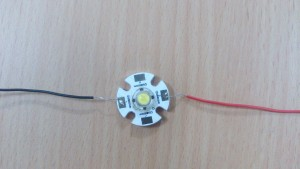# Powering up an LED

Powering up an LED

ledcalc.com ; A useful tool to determine the value of resistors to be used in the circuit.

V = I × R (Ohm’s law)

supply voltage = 5V

resistor used = 2 x 33ohm resistor connected in parallel = 16.5 ohm

to determine current ; I = V / R

5 / 16.5 = 300mA

(Power supply voltage − LED voltage) / current (in amps) = desired resistor value (in ohms)

To calculate the amount of power that the resistor will dissipate;

Power Rule: P = (I × V ) W
If a current I flows through through a given element in your circuit, losing voltage V in the process, then the power dissipated by that circuit element is the product of that current and voltage: P = I × V.## One Reply to “Powering up an LED”

1.Sanjeev says:

1) Give note on approximate voltage of an LED ~2.5V for reasonable current.
2) Give an estimate of back of the envelope calculations
(5V-2.5V)/16.5 ~ 150 mA.
From using the tool what does the same give.
What is the wattage of the LED and resistors.